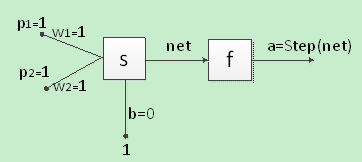【自己动手写神经网络】小白入门连载（三）--神经元的感知 原

【真实原创，转载务必注明出处】

3.1 常用传输函数列表

 P 形状 颜色 苹果 1 1 香蕉 -1 -13.1 感知机识别苹果

=1+1+0

=2

Step(net)=1

net=p1*w1+p2*w2+b

=-1-1+0

=-2

Step(net)=0

wnew=wold+ep

bnew=bold+e

net=p1*w1+p2*w2+b

=1-1+0

=0

Step(net)=0

e=t-a

=1-0

=1

w1new=w1old+ep

=1+1*1

=2

w2new=w2old+ep

=-1+1*1

=0

bnew=bold+e

=0+1

=1

net=p1*w1+p2*w2+b

=1*2+1*0+1

=3

Step(net)=1

net=p1*w1+p2*w2+b

=-1*2-1*0+1

=-1

Step(net)=0

python深度学习大全 原文地址：https://www.zybuluo.com/hanbingtao/note/433855 深度学习是啥 在人工智能领域，有一个方法叫机器学习。在机器学习这个方法里，有一类算法叫神经网络。神经网...

luanpeng825485697
2018/01/09
0
0

机器之心整理 　　参与：机器之心编辑部 　　 　　在今天的 2018 云栖大会·上海峰会上，阿里巴巴发布了免费的天池深度学习课程，涵盖了神经网络基础、CNN、RNN、递归网络、强化学习以及...

2018/06/07
0
0

2017/07/13
0
0

2017/11/09
0
0

imhy
2018/06/18
0
0

Phpstorm2018 永久激活

happyfish319
21分钟前
5
0

###来看一下Android中除了AIDL还有哪些进程间通信的方式： 1、Bundle Bundle实现了Parcelable，所以在Android中我们可以通过Intent在不同进程间传递Bundle数据。 但是在Intent 传输数据的过程...

22分钟前
6
0

Java技术剑
23分钟前
6
0
java 多线程

25分钟前
7
0

29分钟前
4
0